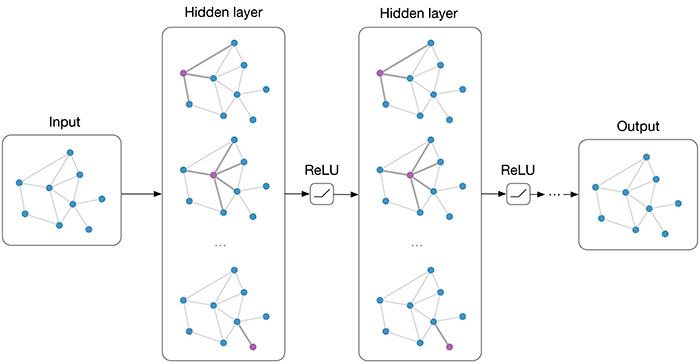## Graph Convolutional Networks

Published: 09 Oct 2015 Category: deep_learning

Learning Convolutional Neural Networks for Graphs

Convolutional Neural Networks on Graphs with Fast Localized Spectral Filtering

Semi-Supervised Classification with Graph Convolutional Networks

Graph Based Convolutional Neural Network

How powerful are Graph Convolutions? (review of Kipf & Welling, 2016)

http://www.inference.vc/how-powerful-are-graph-convolutions-review-of-kipf-welling-2016-2/

Graph Convolutional NetworksDeepGraph: Graph Structure Predicts Network Growth

Deep Learning with Sets and Point Clouds

Deep Learning on Graphs

Robust Spatial Filtering with Graph Convolutional Neural Networks

https://arxiv.org/abs/1703.00792

Modeling Relational Data with Graph Convolutional Networks

https://arxiv.org/abs/1703.06103

Distance Metric Learning using Graph Convolutional Networks: Application to Functional Brain Networks

Deep Learning on Graphs with Graph Convolutional Networks

Deep Learning on Graphs with Keras

Learning Graph While Training: An Evolving Graph Convolutional Neural Network

https://arxiv.org/abs/1708.04675

Graph Attention Networks

Residual Gated Graph ConvNets

https://arxiv.org/abs/1711.07553

Probabilistic and Regularized Graph Convolutional Networks

Videos as Space-Time Region Graphs

https://arxiv.org/abs/1806.01810

Relational inductive biases, deep learning, and graph networks

Can GCNs Go as Deep as CNNs?

GMNN: Graph Markov Neural Networks

DeepGCNs: Making GCNs Go as Deep as CNNs

Rethinking pooling in graph neural networks